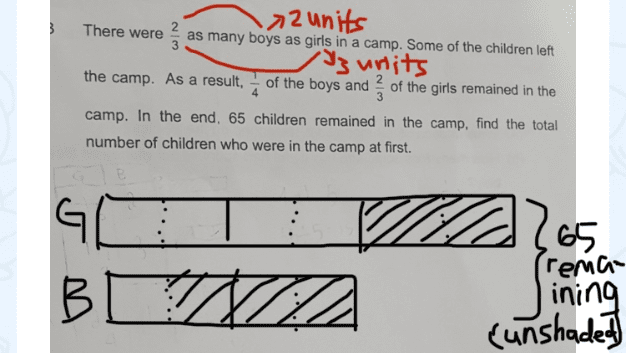# QuestionThank you.

I can only do algebra.  I’ll leave it to others to do the model answer.

Let B be the original number of boys in the camp.
Let G be the original number of girls in the camp.

(1) B = 2/3 * G

(2) 1/4 * B + 2/3 * G = 65

Substituting (1) into (2),
1/4 * B + B = 65
=> 5/4 * B = 65
Therefore B = 52

So, G = 3/2 * 52 = 78

So the total number of children in the camp at first = B + G = 52 + 78 = 130 #

Thank you so much! 🙏

0 Replies 0 LikesDraw the model above based on the question. You have to divide each big block into half as shown by the dotted lines since 3/4 of the boys leave.

Rest of the working:

5 units —> 65

1 unit —> 65 / 5 = 13

10 units —> 130

There were 130 students at first.